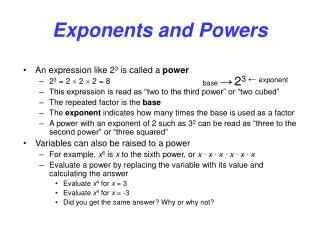Download PresentationExponents and Powers

# Exponents and Powers

Download Presentation## Exponents and Powers

- - - - - - - - - - - - - - - - - - - - - - - - - - - E N D - - - - - - - - - - - - - - - - - - - - - - - - - - -
##### Presentation Transcript

1. Exponents and Powers • An expression like 23 is called a power • 23 = 2  2  2 = 8 • This expression is read as “two to the third power” or “two cubed” • The repeated factor is the base • The exponent indicates how many times the base is used as a factor • A power with an exponent of 2 such as 32 can be read as “three to the second power” or “three squared” • Variables can also be raised to a power • For example, x6 is x to the sixth power, or x ∙x ∙x ∙x ∙x ∙x • Evaluate a power by replacing the variable with its value and calculating the answer • Evaluate x4 for x = 3 • Evaluate x4 for x = -3 • Did you get the same answer? Why or why not? base →23 ←exponent

2. Grouping symbols • Grouping symbols enclose parts of an expression that represent a single number • Parentheses ( ) • Brackets [ ] • Braces { } • Absolute value symbols | | also act as grouping symbols • A fraction bar is another grouping symbol • The numerator and denominator are separate expressions • Grouping symbols affect the result of power expressions • For example • -22 = -4, while (-2)2 = 4 • 1 + 23 = 9, while (1 + 2)3 = 27